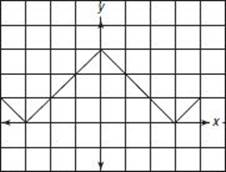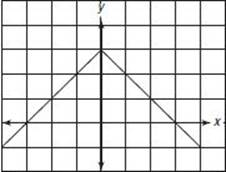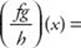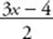﻿ ﻿Exercises - Functions and Graphs - FUNCTIONS - SAT SUBJECT TEST MATH LEVEL 1

## FUNCTIONS## CHAPTER 15Functions and Graphs### Exercises1. If {(1, 5), (2, 13), (x, y)} is a function, which of the following must be true?

I. x > 2

II. y13

III. x1

(A) None

(B) I only

(C) II only

(D) III only

(E) I and II only

2. If f (x)= –3x3, what is the value of f (–8)?

(A) 78

(B) 1,530

(C) 1,542

(D) 13,818

(E) 13,830

3. What is the domain of the function(A) All real numbers

(B) {x | –2 < x < 2}

(C) {x | x2}

(D) {x | x-2}

(E) {x | x < -2 or x > 2}

4. If f(x) = x +, what is f (f (x))?

(A) 1

(B) x =(C) x2 +(D)(E)5. Which of the following graphs is not the graph of a function?

(A)(B)(C)(D)(E)6. What is the range of the function(A) All real numbers

(B) All real numbers greater than or equal to 0

(C) All real numbers greater than or equal to 2

(D) All real numbers greater than or equal to 2 or less than or equal to –2

(E) All real numbers between –2 and 2, inclusive.

7. Which of the following is not the equation of a function?

(A) y = 3

(B) x = 3

(C) y = x

(D) y = |x|

(E) y =Questions 8 and 9 refer to the following graphs. The first one is the graph of y = |x|.y = f (x) = x(A)(B)(C)(D)(E)

8. Which of the graphs is the graph of y = |x – 3|?

(A) Graph A

(B) Graph B

(C) Graph C

(D) Graph D

(E) Graph E

9. Which of the graphs is that of y = ||x|–3|?

(A) Graph A

(B) Graph B

(C) Graph C

(D) Graph D

(E) Graph E

10. If x1, 2, f (x) = x2 – 1, g(x) = x2 – 4, and h(x) = x2 – 3x + 2, then(A) x2 + 3x + 2

(B) x2 – 3x + 2

(C) x4 – 4

(D)(E)11. If f (x) = x2 – 1 and g(x) = x2 + 1, what is (f o g)(x) – (g o f )(x)?

(A) –2

(B) 0

(C) x4 – 1

(D) 4x2

(E) 4x2 – 2

12. If f(x)=, then f –1(x) =

(A)(B)(C)(D)(E)1. (A) 5. (C) 9. (D) 2. (C) 6. (B) 10. (A) 3. (D) 7. (B) 11. (E) 4. (E) 8. (C) 12. (A)

Solutions

Each of the problems in this set of exercises is typical of a question you could see on a Math 1 test. When you take the model tests in this book and, in particular, when you take the actual Math 1 test, if you get stuck on questions such as these, you do not have to leave them out—you can almost always answer them by using one or more of the strategies discussed in the “Tactics” chapter. The solutions given here do not depend on those strategies; they are the correct mathematical ones.

See Important Tactics for an explanation of the symbol ⇒, which is used in several answer explanations.

1. (A) Certainly, x can be 0 or –7 or 1.5, or any number less than 2. (I is false.) If x = 2, then y must be 13. So y can equal 13. (II is false.) If y5, then x cannot be 1; but it is perfectly all right to have x = 1 and y = 5. (III is false.) None of statements I, II, and III is true.2. (C) f (–8) = –3(–8)3 – 3= (–3)(–512) – 3(–2)
= 1,536 + 6 = 1,542

3. (D) The only restriction is that the denominator of f (x) cannot be 0. So x–2.

4. (E)5. (C) Only graph C fails the vertical line test. The line x = 0 crosses graph C twice.

6. (B) The square root of a number cannot be negative, so no negative number is in the range. If b0 and if, then x2 = b 2 + 4 and. So every number greater than or equal to 0 is in the range.

7. (B) The graph of x = 3 is a vertical line that passes through (3, 0), (3, 1), (3, 2), . . . Since there is more than one ordered pair whose first component is 3, it is not the graph of a function. Also, the vertical line x = 3 intersects the line x = 3 more than once—infinitely many times, in fact. All the other choices are functions.

8. (C) By KEY FACT N4, the graph of y = |x – 3| is obtained by shifting the graph of y = |x | three units to the right.

9. (D) By KEY FACT N4, the graph of y = |x | – 3 is obtained by shifting the graph of y = |x | three units down as shown in graph A. The graph of y = ||x | – 3| consists of the reflection in the x-axis of all the points on graph A that are below the x-axis together with all the points on graph A that are on or above the x-axis. The correct graph is D.

10. (A)11. (E)12. (A) WriteSwitch x and y, and solve for y :﻿